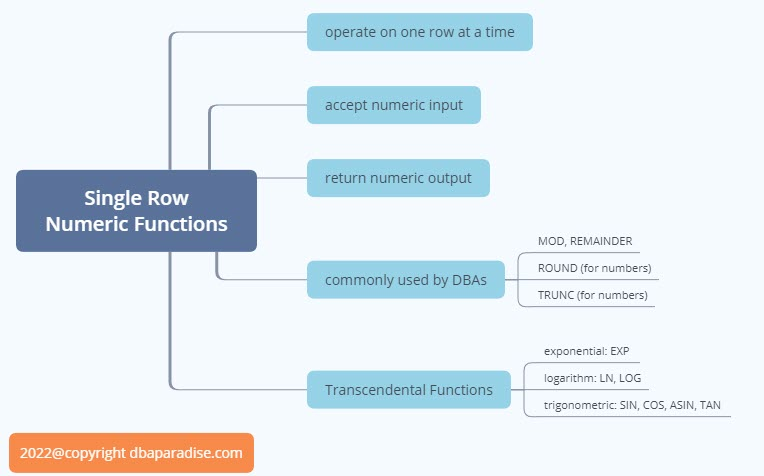# Useful Examples For DBAs of Single Row Numeric Functions

August 26, 2022 Off

In the previous post, we started talking about Single Row Functions, which return a single value (row) for each row of a queried object, basically they operate on one row at a time. Today we will talk about Numeric Functions, and see some examples! Single Row Numeric Functions can be found in the SELECT, WHERE, START WITH and CONNECT BY, HAVING clauses of a select statement.

Numeric Functions accept an input and return a value. Both input and return values are numeric. The return value is usually a NUMBER that can be accurate up to 30-36-38 decimal digits, depending on the actual function.

Transcendental functions are also numberic functions. These include the exponential, logarithm, and trigonometric functions: ACOS, ASIN, COS, SIN, TAN,EXP,LN, LOG and more. As DBAs, we do not use transcendental functions vey much.

Let’s look at numeric functions that are more commonly used by DBAs: MOD, REMAINDER, ROUND, TRUNC.

MOD Function takes two input values, a,b and it returns the remainder of a divided by b. If b is 0 then MOD returns a, as we cannot divide by 0. So MOD(a,b) will return the remainder of a divided by b, and if b is 0 then it will return a.

Where do we use the MOD function?

Usually, we use this function to see if a number is odd or even, or if a number is divisible by another number. Here are some examples of MOD function in the SELECT clause and WHERE clause of a SQL Statement:

1) Return the remainder

```select mod(3,2) as remainder from dual;

REMAINDER
----------
1

```

2) Check if number is odd or even

```select case mod(100,2) when 0 then 'Even' else 'Odd' end as number_type
from dual;

NUMBER_TYPE
-----------
Even
```

3) Check if number is divisible by another number:

```select case mod(100,3)
when 0 then 'Divisible by 3'
else 'Not divisible by 3' end  as number_type from dual;

NUMBER_TYPE
------------------
Not divisible by 3
```

4) Return every second row (or third row) in a result set:

```select last_name, salary, row_number
from ( select last_name, salary, rownum as row_number
from hr.employees order by last_name)
where mod(row_number,2)=0;  --returns every second row
```

5) Return only employees with odd employee number:

```select empno, last_name
from hr.employees
where mod(empno,2)=1;   --returns only odd employee numbers
```

Fun Fact: You can use any numeric value in MOD or REMAINDER functions, not just integers!

```select mod(1.5,2) as remainder from dual;

REMAINDER
---------
1.5

select mod(1.5,1.2) as remainder from dual;

REMAINDER
---------
.3
```

The REMAINDER function is similar to MOD function, the difference is that REMAINDER uses ROUND in the formula, versus MOD is using FLOOR to calculate the values.

ROUND Function, for numbers, returns the input number rounded to the integer places to the right of the decimal point. So ROUND(a,b) returns the number a, rounded to the b number of right decimal point, where b is an integer: round(5.187,1) = 5.2
If b is not specified (aka is 0) then number a is rounded to zero places. The integer b can also be negative, then a is rounded off to the left of the decimal point.

Examples:

```select round(20.4591,3) as example1, round(20.4596,3) as example 2  from dual;

EXAMPLE1  EXAMPLE2
--------  --------
20.459     20.46

select round(20.2591,-2) as example3,round(20.2591,-1) as example4 from dual;

EXAMPLE3  EXAMPLE4
--------  --------
0        20

```

TRUNC function, for numbers, returns the input number truncated to the integer number of decimal places. So TRUNC(a,b) returns the number a, truncated to the b decimal places. If you omit b or b is 0 then a is truncated to 0 places. B can be negative as well, and then the number a will be truncated to b digits left of the decimal point.

Examples:

```select trunc(20.4591,2) as example1, trunc(20.4596,3) as example2 from dual;

EXAMPLE1  EXAMPLE2
--------  --------
20.45    20.459

select trunc(20.4591,-1) as example1, trunc(20.4596,-2) as example2 from dual;

EXAMPLE3  EXAMPLE4
--------  --------
20          0
```